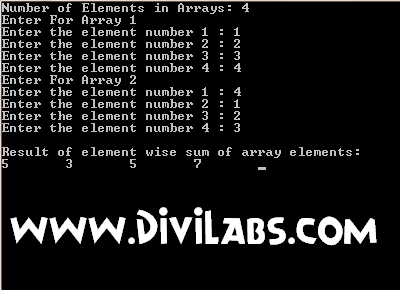# C / C++ Program to calculate element wise sum of 2 arrays : element wise addition

/* The following C / C++ program require user to enter the elements of arrays having equal number of array elements. Equal number of array elements are required in this program. This program can be extended to any of the data type & to the 'n' number of arrays simultaneously*/

Code:
#include<iostream>
#include<conio.h>
using namespace std;
void main ()
{
int long a,b,c;
int d=0,n;
cout<<"Number of Elements in Arrays: ";
cin>>n;
cout<<"Enter For Array 1"<<endl;
while (d<n)
{
cout<<"Enter the element number "<<d+1<<" : ";
cin>>a[d];
d=d+1;
}
d=0;
cout<<"Enter For Array 2"<<endl;
while (d<n)
{
cout<<"Enter the element number "<<d+1<<" : ";
cin>>b[d];
d=d+1;
}
d=0;
while (d<n)
{
c[d]=a[d]+b[d];
d=d+1;
}
d=0;
cout<<"\nResult of element wise sum of array elements:\n";
while (d<n)
{
cout<<c[d]<<"\t";
d=d+1;
}
getche();
}

OutputOutput of C / C++ Program to calculate element wise sum of 2 arrays